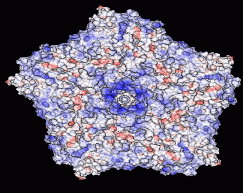## "GEM" -- Analytical Poisson-Boltzmann

Electrostatic interactions are a key factor determining properties of biomolecules. Ability to compute electrostatic potential generated by a molecule is often essential to understand the mechanism behind its biological function such as catalytic activity or ligand binding. To obtain the electrostatic potential everywhere in space, the (linearized) Poisson-Boltzmann equation -- 2nd order PDE -- is usually solved, traditionally by numerical approaches. We are working on a new theory -- ALPB -- which allows one to compute electrostatic potential around biomolecules orders of magnitude faster (and with much less memory needed) than the traditional approach based on numerical solution of the PB equation. A software package GEM has been developed, mainly by John Gordon, to visualize and manipulate electrostatic potentials. Remarkably, it was the ability to visualize and compare approximate analytical potentials with the exact and numerical references that allowed us (this part is mostly due to Andrew Fenley), after many a trial and error, to understand and capture the key physics of the problem in the form of a very simple analytical formula.The new model...... can be used to quickly estimate electrostatic potential around gigantic structures such as viruses. Shown here is the computed potential inside the putative RNA binding pocket on the inner surface of a viral capsid (Tobacco Ring Spot Virus).

### Changes of electrostatic potential on the surface of TRSV viral capsid as a function of pH of the environment. A WMV Movie. 1.6 Mb (if you are using a browser other than MS Internet Explorer, right-click save as)

The outer surface of the TRSV viral capsid is color-coded according to the computed electrostatic potential on its surface. The charge state of the capsid changes with the pH of the environment: computations are performed at a constant salt concentration (0.15 M) and three different pH values shown under each structure. Continuous color scale is used, from red (corresponding to -4.68 kcal/mol/|e|), to blue (+4.68 kcal/mol/|e|). The regions of zero potential are shown in white. The arrow points to an outcropping that corresponds to the center of the pentamer shown in the figure.

E-mail: onufriev@cs.vt.edu